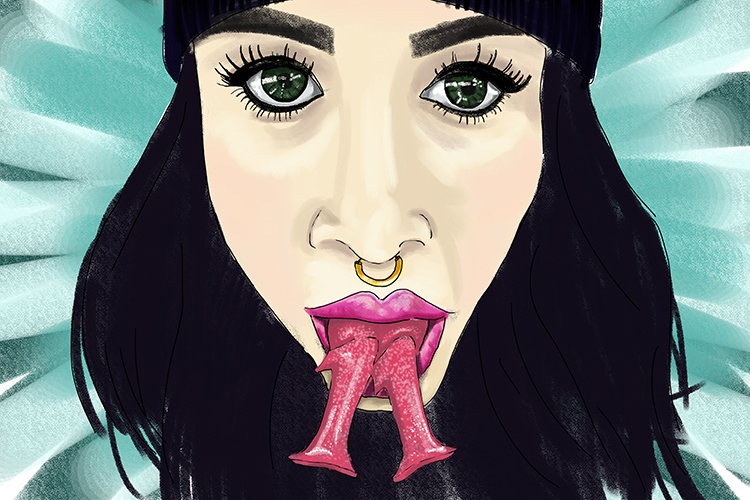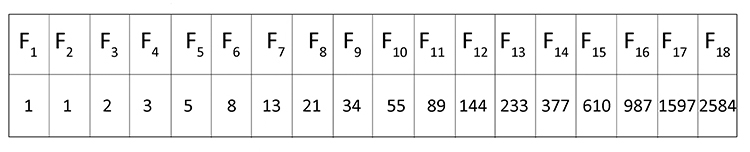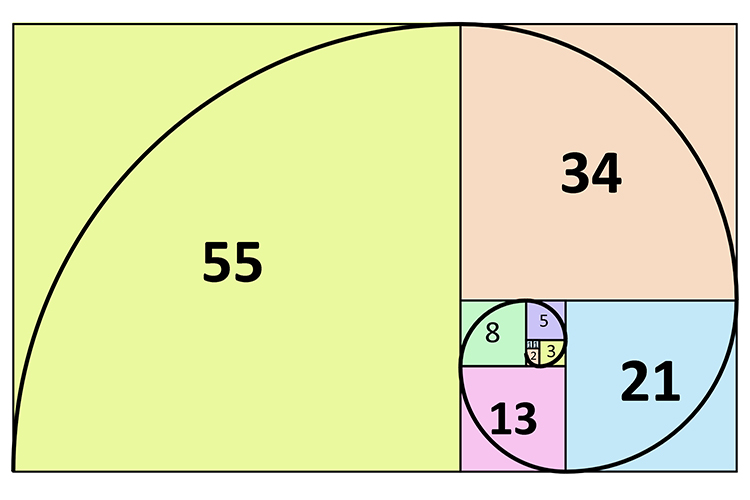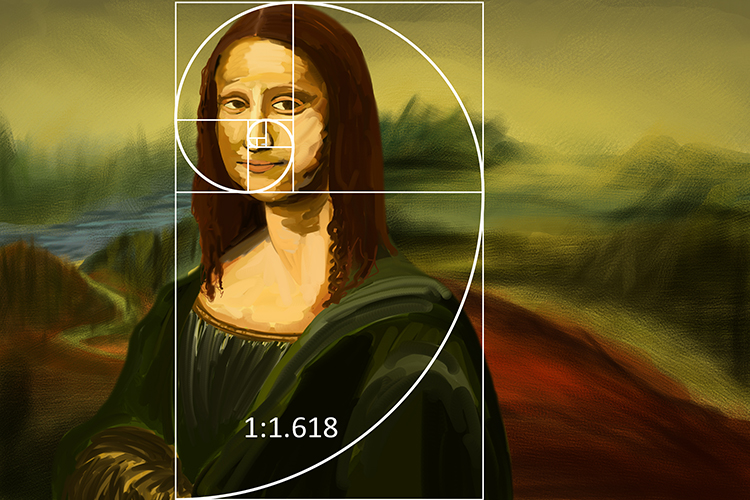# Fibonacci sequence

Fibonacci sequence – A series of numbers in which each number (Fibonacci number) is the sum of the two preceding numbers. The simplest is; 1, 1, 2, 3, 5, 8 etcIf you fib, you lie and are said to have a forked tongue, which looks like two number 1’s next to each other which is the simplest start of the Fibonacci number.

Example 1Example 2The Fibonacci sequence can be used to produce a Fibonacci spiral as shown above. This spiral is used by artists as a close approximation to the golden ratio when composing artwork.The Fibonacci spiral is used by artists to create natural proportions and position elements within the image in order to draw the viewers eye to areas the artists wants to emphasise.